# ∈-NFA of Regular Language L = (0+1)*(00 + 11) and L = b + ba*

• Difficulty Level : Easy
• Last Updated : 05 Jan, 2021

Non-Deterministic Finite Automata and ∈-Non-Deterministic Finite Automata are almost the same except for their transition function and there are a few special rules for construction of ∈-NFA.

```∈-NFA is defined in 5 tuple representation {Q, q0, Σ, δ, F}
where -
Q is the set of all states,
q0 is the initial state,
Σ is the set of input symbols,
δ is the transition function which is δ:Q × (Σ∪∈)->2Q and
F is the set of final states.
```

Simple rules for construction of ∈-NFA :
∈-NFA for a+ :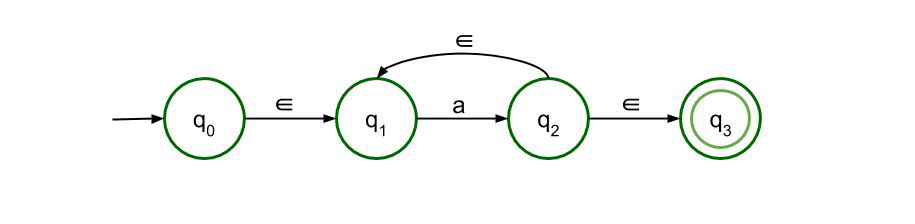This structure is for a+ which means there must be at least one ‘a’ in the expression. It is preceded by epsilon and also succeeded by one. There is epsilon feedback from state q2 to q1 so that there can be more than one ‘a’ in the expression.

∈-NFA for a* :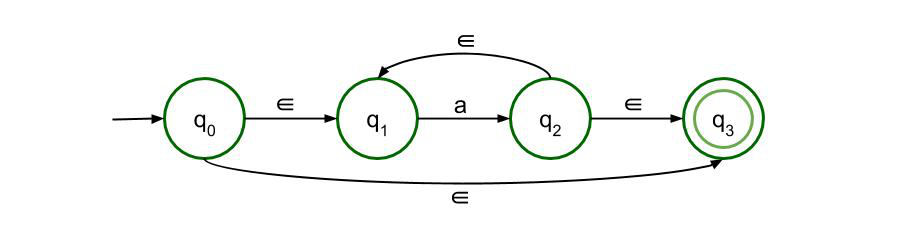This structure is for a* which means there can be any number of ‘a’ in the expression, even 0. The previous structure is just modified a bit so that even if there is no input symbol, i.e. if the input symbol is null, then also the expression is valid.

∈-NFA for a+b :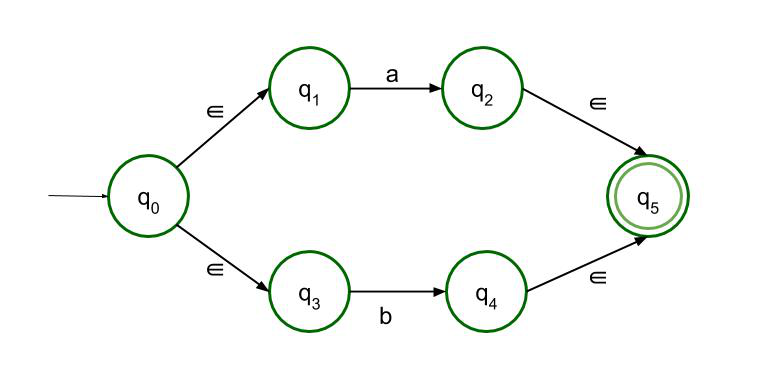This structure accepts either a or b as input. So there are two paths, both of which lead to the final state.
∈-NFA for ab :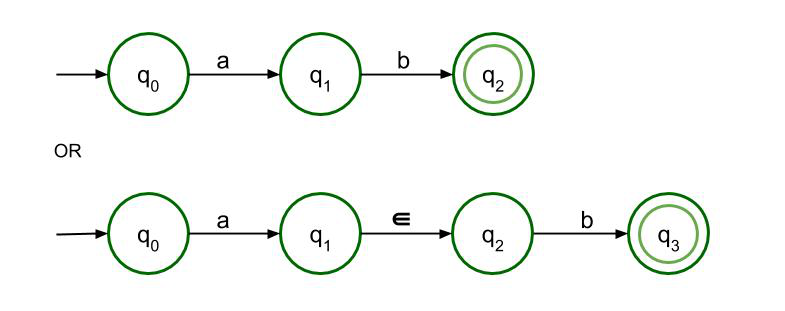For concatenation, a must be followed by b. Only then it can reach the final state. Both structures are allowed here but as it is ∈-NFA so the second structure is recommended.

∈-NFA of Regular Language L = (0+1)*(00 + 11) :
Following the above-mentioned rules, ∈-NFA of Regular Language L = (0+1)*(00 + 11) is to be constructed.
L = (0+1)*(00 + 11) can be divided into two parts – (0+1)* and (00 + 11). Since they are concatenated, the two parts will be linearly connected to each other.

• The first part can be drawn using the third rule and the second rule. (0+1) is easy to draw following the third rule and considering (0+1) as one unit, (0+1)* can also be drawn applying the second rule.

Here’s the first part as follows.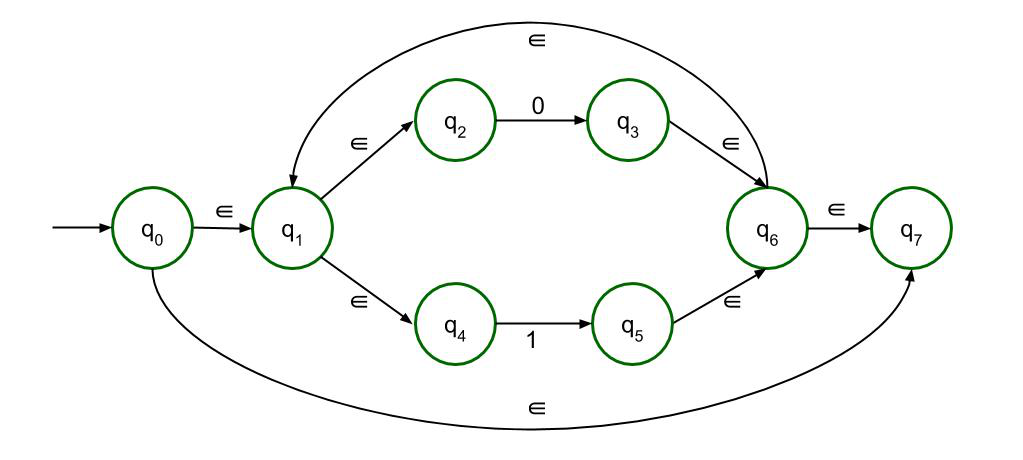• The second part can be drawn with the help of fourth rule. In the fourth rule, a and b both are 0. That is how we construct 00. Similarly, we can construct 11. now since they are connected by ‘+’ sign, there will be two paths connecting both these structures.

Here’s the second part as follows.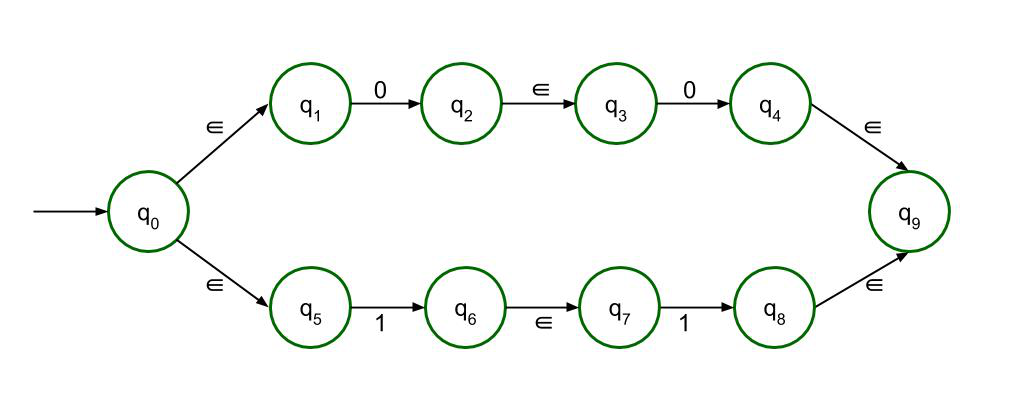The Final ∈-NFA will be :
Connecting the two structures linearly gives us our final ∈-NFA.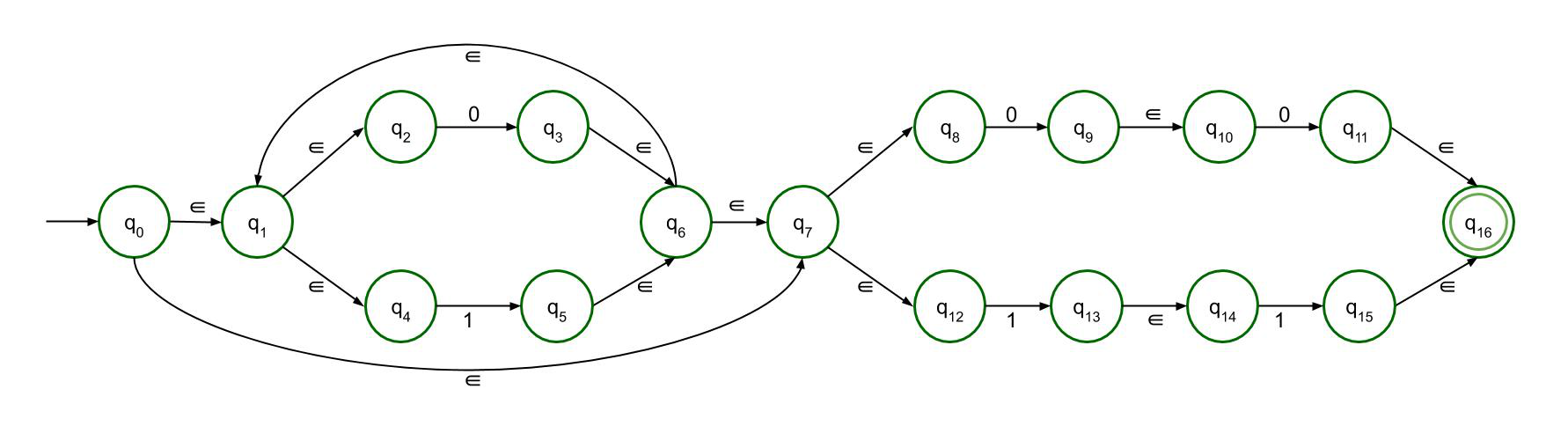∈-NFA of Regular Language L = b + ba* :
Following the above-mentioned rules, ∈-NFA of Regular Language L =b + ba* is to be constructed.
L =b + ba* has two terms. The first term is fairly easy to construct. Since both the terms are connected by ‘+’ sign, there will be two paths coming out of the first node. The second term is to be drawn following the second rule of construction, a* which is simply preceded by b.

The Final ∈-NFA will be :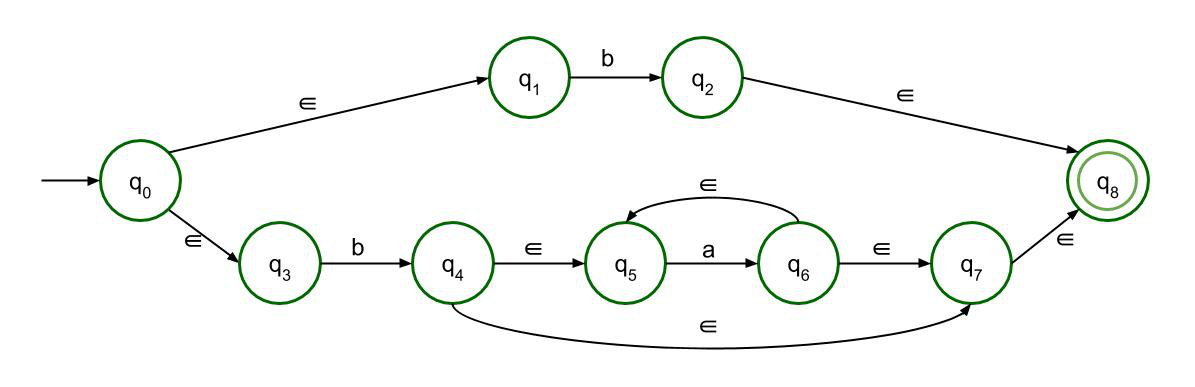My Personal Notes arrow_drop_up